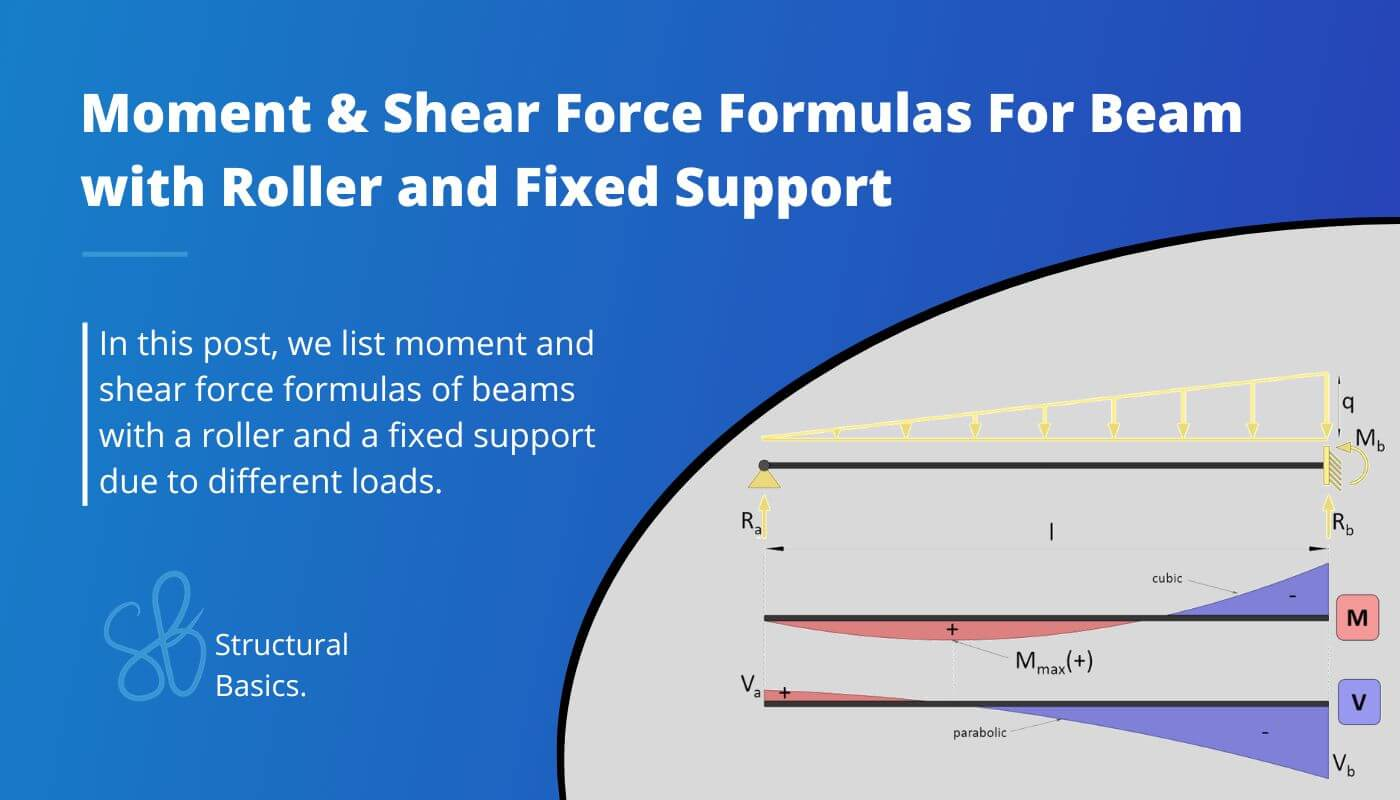# Beam With Fixed And Roller Support -Moment And Shear Force Formulas Due To Different LoadsCalculating bending moments and shear forces in beams – in this case beams with one fixed and one roller support – for different loading scenarios, is probably one of the things in structural engineering that we do throughout our studies and also careers later on.

While it’s very important to know how to derive and calculate the internal forces, the further we get in our studies, the more we can use beam moment and shear formulas.

After having covered the moment and shear formulas for simply supported and cantilever beams, in this article, we’ll show, the most important and easiest formulas for beams with one fixed and one roller support due to different loading scenarios like UDL line loads, point loads, external moments and triangular loads.

All will be shown and explained by examples.

Here is a quick overview of what we cover in this post.

Now, before we get started, always remember that the unit of the bending moment is Kilonewton meter [$kNm$] and Kilonewton [$kN$] for the shear forces when in Europe.

But now, let’s get started.

## 1. Uniformly distributed line load (UDL) formulas

### Bending moment

$M(x) = 1/8 \cdot q \cdot x \cdot (3l-4x)$

### Max positive bending moment

$M_{max} = 9/128 \cdot q \cdot l^2$

### Max negative bending moment (at support b)

$M_{max} = -1/8 \cdot q \cdot l^2$

### Shear force at support a

$V_{a} = 3/8 \cdot q \cdot l$

### Shear force at support b

$V_{b} = -5/8 \cdot q \cdot l$

### Reaction force support a

$R_a = 3/8 \cdot q \cdot l$

### Reaction force support b

$R_b = 5/8 \cdot q \cdot l$

## 2. Uniformly distributed line load (UDL) at left support (formulas)

### Max positive bending moment

$M(a) = 1/8 \cdot q \cdot a^2 \cdot (4-6\cdot \frac{a}{l} + (\frac{a}{l})^3)$

### Max negative bending moment (at support b)

$M_{b} = -1/8 \cdot q \cdot a^2\cdot (2- (\frac{a}{l})^2)$

### Shear force at support a

$V_{a} = 1/8 \cdot q \cdot a \cdot (8-6\cdot \frac{a}{l} + (\frac{a}{l})^3)$

### Shear force at support b

$V_{b} = -1/8 \cdot q \cdot a\cdot (6\cdot \frac{a}{l} – (\frac{a}{l})^3)$

### Reaction force support a

$R_{a} = 1/8 \cdot q \cdot a \cdot (8-6\cdot \frac{a}{l} + (\frac{a}{l})^3)$

### Reaction force support b

$R_b = 1/8 \cdot q \cdot a\cdot (6\cdot \frac{a}{l} – (\frac{a}{l})^3)$

## 3. Point load at midspan (formulas)

### Max positive bending moment

$M_{max} = 5/32 \cdot Q \cdot l$

### Max negative bending moment (at support b)

$M_{b} = -3/16 \cdot Q \cdot l$

### Shear force at support a

$V_{a} = 5/16 \cdot Q$

### Shear force at support b

$V_{b} = -11/16 \cdot Q$

### Reaction force support a

$R_{a} = 5/16 \cdot Q$

### Reaction force support b

$R_b = 11/16 \cdot Q$

## 4. Intermediate point load (formulas)

### Max positive bending moment

$M(a) = 1/2 \cdot Q \cdot a \cdot (\frac{b}{l})^2 \cdot (2 + \frac{a}{l})$

### Max negative bending moment (at support b)

$M_{b} = -1/2 \cdot Q \cdot a \cdot (1 – (\frac{a}{l})^2)$

### Shear force at support a

$V_{a} = 1/2 \cdot Q \cdot (\frac{b}{l})^2 \cdot (2 + \frac{a}{l})$

### Shear force at support b

$V_{b} = -Q \cdot \frac{a}{2l} \cdot (3 – (\frac{a}{l})^2)$

### Reaction force support a

$R_{a} = 1/2 \cdot Q \cdot (\frac{b}{l})^2 \cdot (2 + \frac{a}{l})$

### Reaction force support b

$R_b = Q \cdot \frac{a}{2l} \cdot (3 – (\frac{a}{l})^2)$

## 5. Triangular load 1 (formulas)

### Bending moment

$M(x) = 1/2 \cdot q \cdot l \cdot x \cdot (\frac{1}{5} – \frac{1}{3} \cdot (\frac{x}{l})^2)$

### Max positive bending moment

$M_{max} = 0.0298 \cdot q \cdot l^2$ at x = 0.447 l

### Max negative bending moment (at support b)

$M_{b} = -1/15 \cdot q \cdot l^2$

### Shear force at support a

$V_{a} = 1/10 \cdot q \cdot l$

### Shear force at support b

$V_{b} = -2/5 \cdot q \cdot l$

### Reaction force support a

$R_{a} = 1/10 \cdot q \cdot l$

### Reaction force support b

$R_b = 2/5 \cdot q \cdot l$

## 6. Triangular load 2 (formulas)

### Bending moment

$M(x) = 1/2 \cdot q \cdot l \cdot x \cdot (\frac{11}{20} – \frac{x}{l} + \frac{1}{3}\cdot (\frac{x}{l})^2)$

### Max positive bending moment

$M_{max} = 0.0424 \cdot q \cdot l^2$ at x = 0.33 l

### Max negative bending moment (at support b)

$M_{b} = -7/120 \cdot q \cdot l^2$

### Shear force at support a

$V_{a} = 11/40 \cdot q \cdot l$

### Shear force at support b

$V_{b} = -9/40 \cdot q \cdot l$

### Reaction force support a

$R_{a} = 11/40 \cdot q \cdot l$

### Reaction force support b

$R_b = 9/40 \cdot q \cdot l$

If you are new to structural design, then check out our design tutorials where you can learn how to use the calculated bending moments and shear forces to design structural elements such as

Do you miss any Moment or shear force formulas for this beam with fixed and hinge support that we forgot in this article?

Let us know in the comments✍️